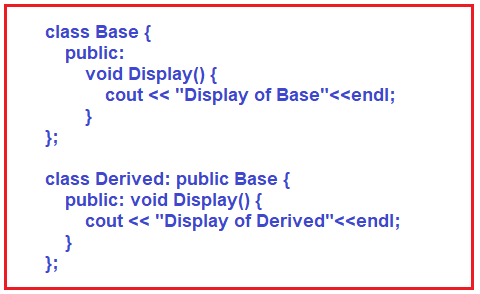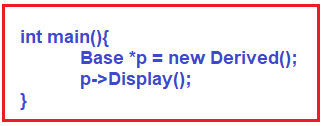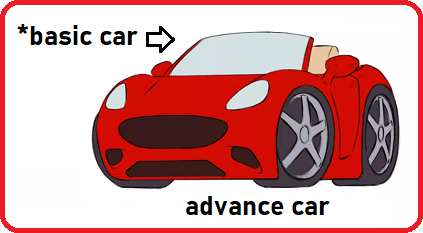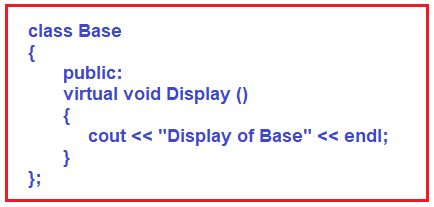# Virtual Functions in C++

## Virtual Functions in C++ with Examples:

In this article, I am going to discuss Virtual Functions in C++ with Examples. Please read our previous article where we discussed Function Overriding in C++ with Example.

##### What are Virtual Functions in C++?

A virtual function in C++ is a member function that is declared within a base class using the virtual keyword and is re-defined by a derived class. When we refer to a derived class object using the base class pointer or base class reference variable, and when we can call a virtual function, then it will execute the function from the derived class. So, Virtual Functions in C++ ensure that the correct function is called for an object, regardless of the expression used to make the function call.

##### Example to Understand Virtual Functions in C++:

Let us understand the need for Virtual Functions in C++ with an example. Please have a look at the following code. Here, we have a base class having one function called Display and it will print “Display of Base”. And also, we have a derived class having function Display which will display “Display of Derived” and this class is deriving from the Base class.We already discussed the concept of Base Class Pointer pointing to the Derived Class Object in one of our Previous Articles with Examples. Let us do it.Inside the main function, we have taken a base class pointer p and to that, we have assigned the Derived class object. Then which functions we can call by using the base class pointer? Only those functions are present in the base class. So, pointer ‘p’ has called the Display function. But this function is overridden in the Derived class also. So, there are two copies now.

Now the question is whose function will be called, base class function or derived class function? See base class is having a function and the Derived class has overriding that function, base class pointer, and derived class object and calls a function that is present in both. So, whose function will be called? The base class function will be called. The complete example code is given below.

```#include <iostream>
using namespace std;

class Base
{
public:
void Display ()
{
cout << "Display of Base" << endl;
}
};

class Derived:public Base
{
public:void Display ()
{
cout << "Display of Derived " << endl;
}
};

int main()
{
Base *p = new Derived ();
p->Display ();
}
```

Output: Display of Base

The function will be called based on the pointer that we have used. So, using a base class pointer, when we call an override function then the base class function will be called. Remember this point. This is what happens in C++. Now, let us take the example of real-life and understand it.

##### Real-Time Example to Understand the need for Virtual Function:

Suppose we have a basic car pointer that is pointing to an advanced car object,Pointer is the basic car but the actual object is the advanced car. So, I am thinking of it as a basic car. How can I drive? How it will run? Is it will run like what I am thinking or run like an advanced car? It will run like an advanced car because I am pointing at the object of an advanced car.

So, when you call a function that is present in the base case as well as the derived class, whose function must be called? Based on the object the function must be called. Not based on the pointer.

See it is just like if you saw a donkey and say it’s a horse, will it run like a horse? You are thinking of it like a horse but that’s a donkey. It cannot run like a horse. In the same way, here the basic car is the reference and you are pointing at the advanced car object. So, the object is of the derived class and the pointer is of the base class.

When we call a function then the base class function is called. But logically it is wrong. Whose function must be called? The function must be called based on the object. But, in C++ this doesn’t happen. In C++, if you call a donkey as a horse and say run then it will run like a horse. That’s the meaning.

Now let us see how to make the function of the derived class execute and not the base class in C++. So, for this to happen, C++ is having a keyword called Virtual. Inside the base class, you have to make the function as virtual as shown in the below image.When you override it in the derived class, if you have a base class pointer pointing to the derived class object and when you call the function through the base class pointer, then it will execute the function from the derived class. So, this is what virtual functions are meant for.

For a better understanding, please have a look at the below example. In the below example, we have marked the base class Display function as virtual and then override the function in the Derived class. And then we create the Base class pointer pointing to the Derived class object and invoke the Display method and this time the Display function is going to execute from the Derived class.

```#include <iostream>
using namespace std;

class Base
{
public:
virtual void Display ()
{
cout << "Display of Base" << endl;
}
};

class Derived:public Base
{
public:
void Display ()
{
cout << "Display of Derived" << endl;
}
};

int main()
{
Base *p = new Derived();
p->Display();
}
```

Output: Display of Derived

##### What is Virtual Function in C++?

So, to give real-life meaning to the code, virtual functions are introduced in C++. If you make a base class function as virtual and override it in the derived class, then if you use the base class pointer or base class reference variable and derived class object and call the override method then the derived class function will be called.

If we create an object of derived class d and call the function as ‘d.Display()’ then which function will be called? The derived function will be called. There’s no question here. So, the ambiguity is arising when you have a pointer of one class and the object of another class.

So, to make things work just like in the real world you have to declare virtual functions. So finally, we can say that C++ allows two things to happen, don’t make virtual then the base class function will be called and if we make the function virtual then the derived function will be called. So, everything is in the hands of programmers now. Whether you want to make it virtual or not is the decision of a programmer. Let him decide and do it. So, C++ allows both non-virtual and virtual. So that’s it. This is the concept behind the virtual function and the mechanism of virtual function.

##### Key points of Virtual Functions:
1. Virtual functions are used for achieving polymorphism
2. The base class can have virtual functions
3. Virtual functions can be overrides in the derived class
4. Pure virtual functions must be overrides by the derived class
##### Real-Time Example to Understand Virtual Function in C++:
```#include <iostream>
using namespace std;

class BasicCar
{
public:
virtual void start()
{
cout << "BasicCar started" << endl;
}
};

{
public:void start()
{
cout << "AdvanceCar Started" << endl;
}
};

int main()
{
p->start ();
return 0;
}
```